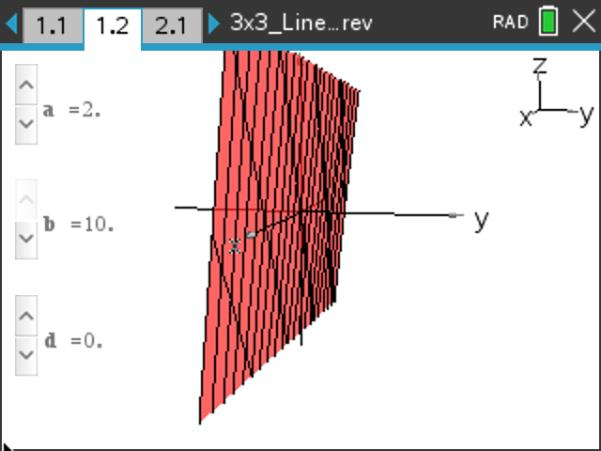# Activities

••• ##### Subject Area

• Math: Precalculus: Other Topics: Matrices, Sequences, and Series

• ##### Author9-12

45 Minutes

• ##### Device
•TI-Nspire™ CX/CX II
•TI-Nspire™ CX CAS/CX II CAS
• TI-Nspire™ Navigator™
• ##### Software

TI-Nspire™ CX
TI-Nspire™ CX CAS

5.0

• ##### Report an Issue

3x3 Linear Systems of Equations#### Activity Overview

This lesson involves connecting graphical representations of systems of linear equations in three variables to the number of solutions of those systems.

#### Objectives

• Students will be able to describe the effects of the coefficients of a linear function in three variables on the graph of the function.
• Students will be able to identify the number of solutions to a system of linear equations in three variables by analyzing the graphs of the equations.
• Students will be able to describe the conditions under which a system of linear equations in three variables will have 0, 1, or infinitely many solutions.

#### Vocabulary

• system of equations
• plane
• solution to a system of equations